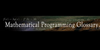### Posynomialis a posynomial. Posynomials are not the same as polynomials in several independent variables. A polynomial`s exponents must be non-negative integers, but its independent variables and coefficients can be arbitrary real numbers; on the other hand, a posynomial`s exponents can be arbitrary real numbers, but its independent variables and coefficie.....
Found on http://en.wikipedia.org/wiki/Posynomial

### PosynomialA positive sum of monomials: Sum_i{c(i)Product_j[x(j)^a(i,j)]}, where c > 0. Each monomial is the product, Product_j[x(j)^a(i,j)] = x(1)^a(i,1) * x(2)^a(i,2) *...* x(n)^a(i,n), and [a(i, j)] is called the exponent matrix. This is the fundamental function in geometric programming. Example: x(1)x(2) + 2x(3)/x(1)^2 is a posynomial with 2 monomial ...
Found on http://glossary.computing.society.informs.org/index.php?page=P.html
No exact match found